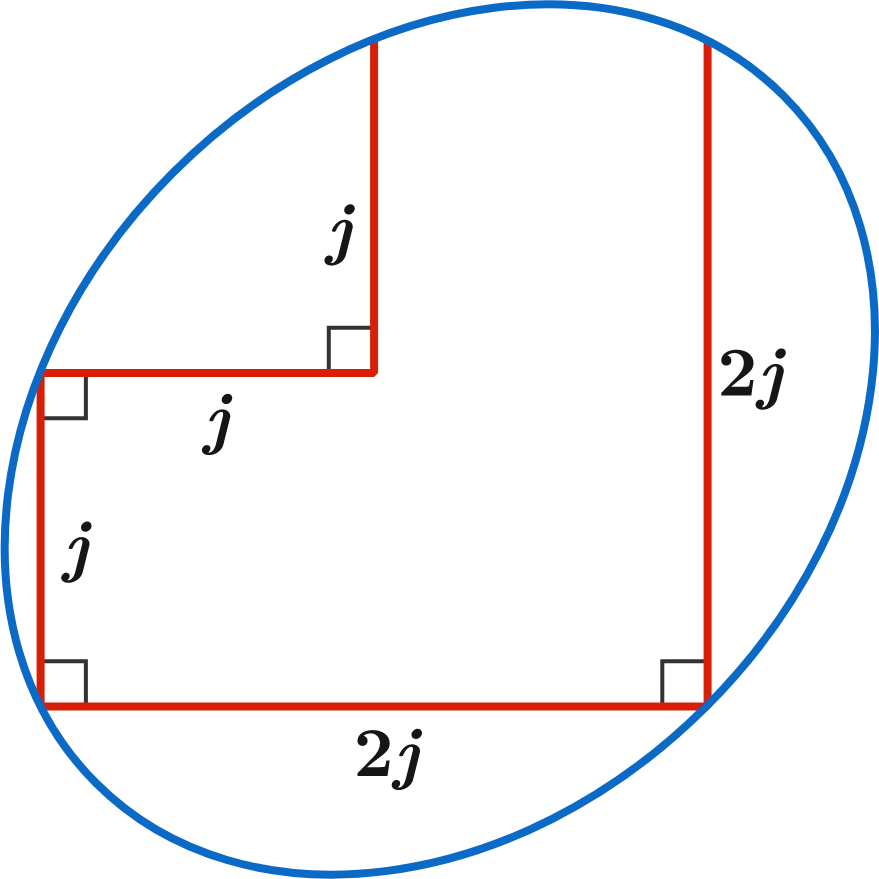Elliptic Mania!

Geometry Level 2In the ellipse shown, let $a$ and $b$ be the lengths of the semi-major and semi-minor axes, respectively.

What is the value of $j$ for which $a^2 + b^2 = 19200?$

×